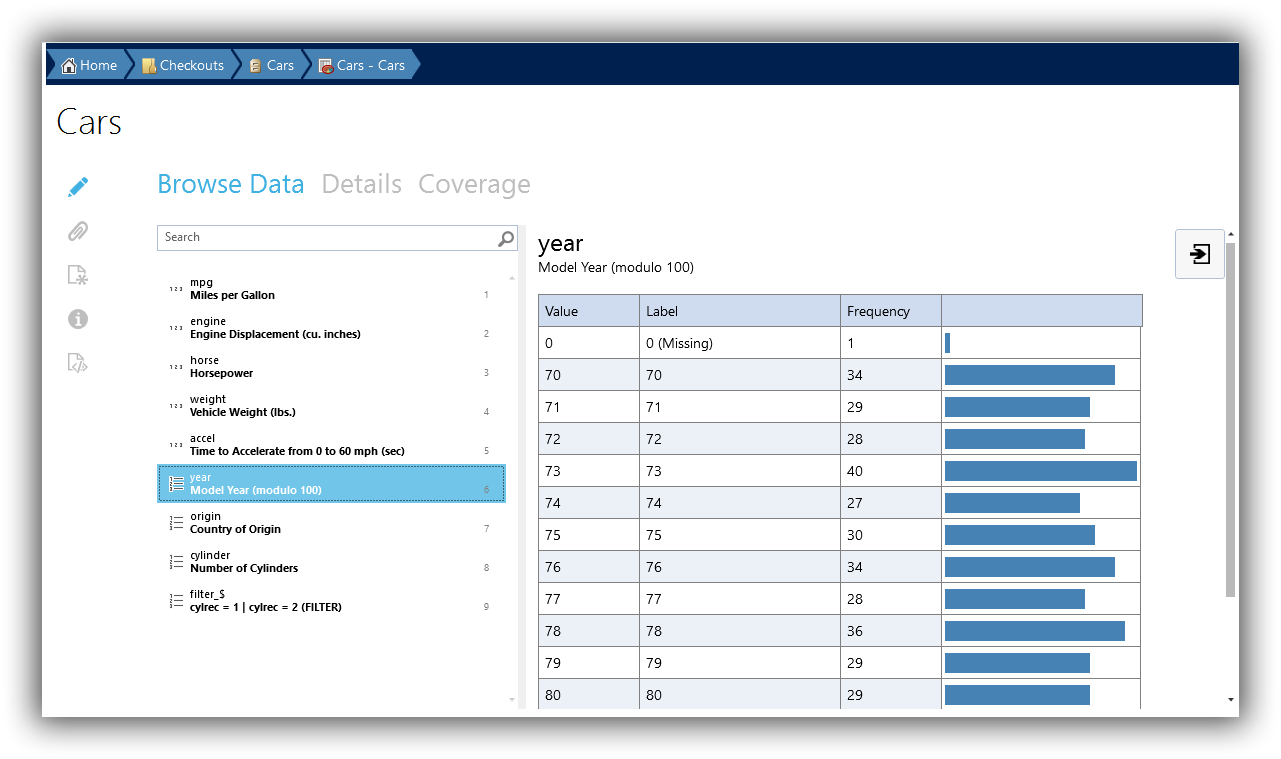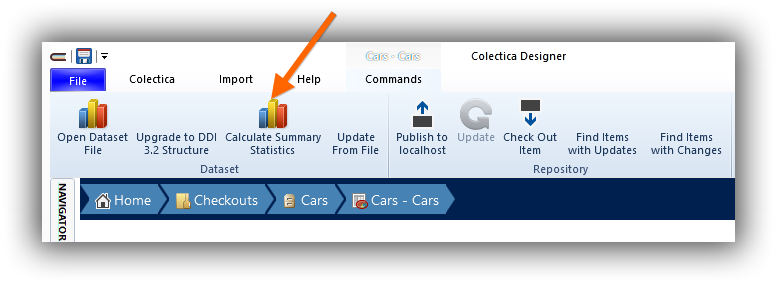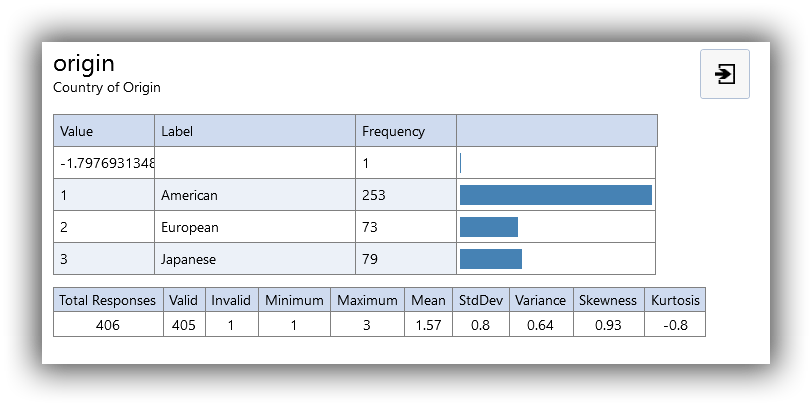# Compute Summary Statistics¶

Colectica can generate descriptive statistics and store this summary information directly in the open standards-based metadata. Supported statistics include:

• Total row count

• Valid

• Invalid

• Category frequencies

• Mean

• Minimum

• Maximum

• Standard deviation

• Variance

• Skewness

• Kurtosis

Optionally, the following statistics can also be calculated. These statistics may take more time and require more memory for larger datasets.

• Median

• Quartiles

To calculate summary statistics for your dataset, follow these steps.

1. Navigate to a dataset.2. On the ribbon, click Calculate Summary Statistics.3. If appropriate, select whether to calculate unweighted and/or weighted statistics.

4. Colectica will automatically calculate summary statistics for each variable in your dataset.

5. When complete, select a variable to view its summary statistics in the details pane.